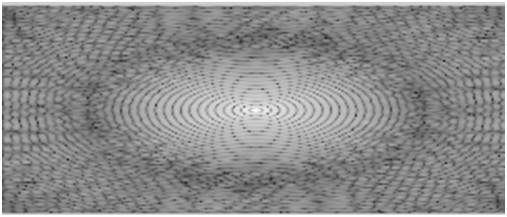# Fourier Series and Transform

In the last tutorial of Frequency domain analysis, we discussed that Fourier series and Fourier transform are used to convert a signal to frequency domain.

## Fourier

Fourier was a mathematician in 1822. He give Fourier series and Fourier transform to convert a signal into frequency domain.

### Fourier Series

Fourier series simply states that, periodic signals can be represented into sum of sines and cosines when multiplied with a certain weight.It further states that periodic signals can be broken down into further signals with the following properties.

• The signals are sines and cosines
• The signals are harmonics of each other

It can be pictorially viewed as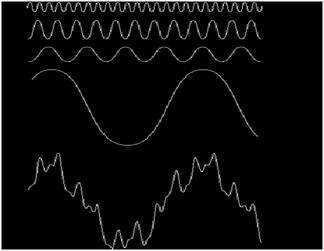In the above signal, the last signal is actually the sum of all the above signals. This was the idea of the Fourier.

### How it is calculated

Since as we have seen in the frequency domain, that in order to process an image in frequency domain, we need to first convert it using into frequency domain and we have to take inverse of the output to convert it back into spatial domain. That’s why both Fourier series and Fourier transform has two formulas. One for conversion and one converting it back to the spatial domain.

### Fourier series

The Fourier series can be denoted by this formula.The inverse can be calculated by this formula.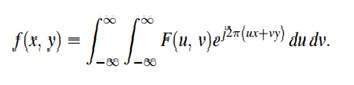## Fourier transform

The Fourier transform simply states that that the non periodic signals whose area under the curve is finite can also be represented into integrals of the sines and cosines after being multiplied by a certain weight.

The Fourier transform has many wide applications that include, image compression (e.g JPEG compression), filtering and image analysis.

## Difference between Fourier series and transform

Although both Fourier series and Fourier transform are given by Fourier , but the difference between them is Fourier series is applied on periodic signals and Fourier transform is applied for non periodic signals

### Which one is applied on images

Now the question is that which one is applied on the images , the Fourier series or the Fourier transform. Well, the answer to this question lies in the fact that what images are. Images are non – periodic. And since the images are non periodic, so Fourier transform is used to convert them into frequency domain.

### Discrete fourier transform

Since we are dealing with images, and in fact digital images, so for digital images we will be working on discrete fourier transformConsider the above Fourier term of a sinusoid. It include three things.

• Spatial Frequency
• Magnitude
• Phase

The spatial frequency directly relates with the brightness of the image. The magnitude of the sinusoid directly relates with the contrast. Contrast is the difference between maximum and minimum pixel intensity. Phase contains the color information.

The formula for 2 dimensional discrete Fourier transform is given below.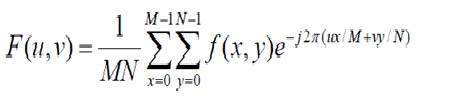The discrete Fourier transform is actually the sampled Fourier transform, so it contains some samples that denotes an image. In the above formula f(x,y) denotes the image, and F(u,v) denotes the discrete Fourier transform. The formula for 2 dimensional inverse discrete Fourier transform is given below.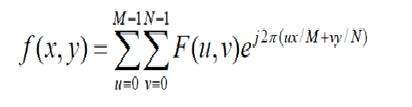The inverse discrete Fourier transform converts the Fourier transform back to the image

### Consider this signal

Now we will see an image, whose we will calculate FFT magnitude spectrum and then shifted FFT magnitude spectrum and then we will take Log of that shifted spectrum.

### Original Image### The Fourier transform magnitude spectrum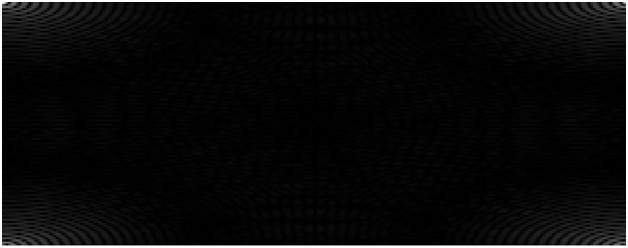### The Shifted Fourier transform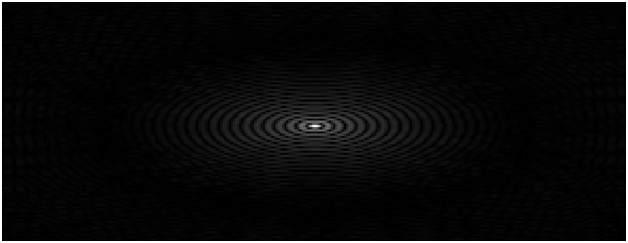### The Shifted Magnitude Spectrum